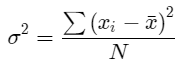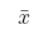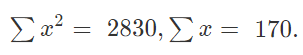# How To Find Variance

Statistics normally means taking the values of some quantities and by plotting it or by arranging it in a meaningful manner. It gives us the process by which we can collect, analyze, interpret, present and organize data. Variance is an important topic in statistics. Variance is a measure of central dispersion. It is the average of the squared difference from the mean. Mean is the average of a given set of numbers. In this article, we will discuss the steps to find variance.

## What is Variance?

The average of the squared differences from the mean is known as variance.

Variance is denoted by σ2.

## How to calculate variance step by step?

You can use the following steps to calculate variance.
Step 1. Calculate the mean of the given numbers.

Step 2. Then for each number, subtract the mean and find the square of the difference.

Step 3. Calculate the average of those squared differences.

## Formulax1, x2, …xn.are the n observations. Mean is denoted by.

Example 1

The means of five observations is 4 and their variance is 5.2. If three of these observations are 1, 2 and 6, then the other two are

(A) 2 and 9

(B) 3 and 8

(C) 4 and 7

(D) 5 and 6

Solution:

Given no. of observations = 5

Mean = 4

Variance = 5.2Let unknown values be a and b.

[(1-4)2 + (2-4)2 + (6-4)2 + (a-4)2 + (b-4)2 ]/5= 5.2

(-3)2 + 22 + 22 + (a-4)2 + (b-4)2 = 26

9 + 4 + 4 + (a-4)2 + (b-4)2 = 26

(a-4)2 + (b-4)2 = 26 – 17

(a-4)2 + (b-4)2 = 9

Substitute the given options in the above equation.

We find that 4 and 7 satisfy the above equation.

Hence option C is the answer.

Example 2

The variance of the first 50 even natural numbers is:

(A) 833/4

(B) 833

(C) 437

(D) 437/4

Solution:

The first 50 even natural numbers are 2, 4, 6,…100.

Variance is given by= [(22+42+…1002)/50 ] – [(2+4+6+…100)/50]2

We will split this and solve it.

(22+42+…1002)/50 = 22(12 + 22+32+…502)/50

Use the formula n(n+1)(2n+1)/6 to find the sum of squares of natural numbers.

22(12 + 22+32+…502)/50 = (22/50)(50×51×101)/6

= 3434

[(2+4+6+…100)/50]2 = [2(1+2+3+..+50)/50]2 {use the formula (n/2)(2a+(n-1)d) to find sum of n natural numbers.}

= [(2/50)(50/2)(2+49)]2

= 512

= 2601

σ 2 = 3434-2601 = 833

Hence option (B) is the answer.

Example 3

In an experiment with 15 observations on x, the following results were availableOn observation that was 20, was found to be wrong and was replaced by the correct value 30. Then the corrected variance is

(A) 78.00

(B) 188.66

(C) 177.33

(D) 8.33

Solution:

Given Σx = 170

Σx2 = 2830

n = 15

20 was replaced by 30. So change in Σx = 10

New Σx = 170+10 = 180

New Σx2 = 2830+302-202

= 2830 + 900 – 400

= 2830 + 500

= 3330

New variance = (3330/15) – (180/15)2

= 222 -122

= 222 – 144

= 78

Hence option (A) is the answer.